Question

# -... Find the transfer function H(w) with the Bode magnitude plot shown in Fig. 14.74 H...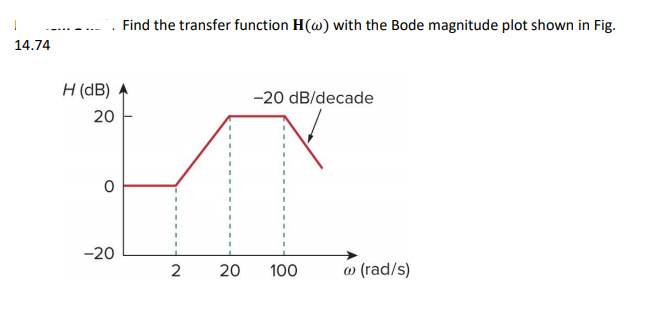-... Find the transfer function H(w) with the Bode magnitude plot shown in Fig. 14.74 H (dB)参 20 20 dB/decade 0 -20L 2 20 100 a) (rad/s)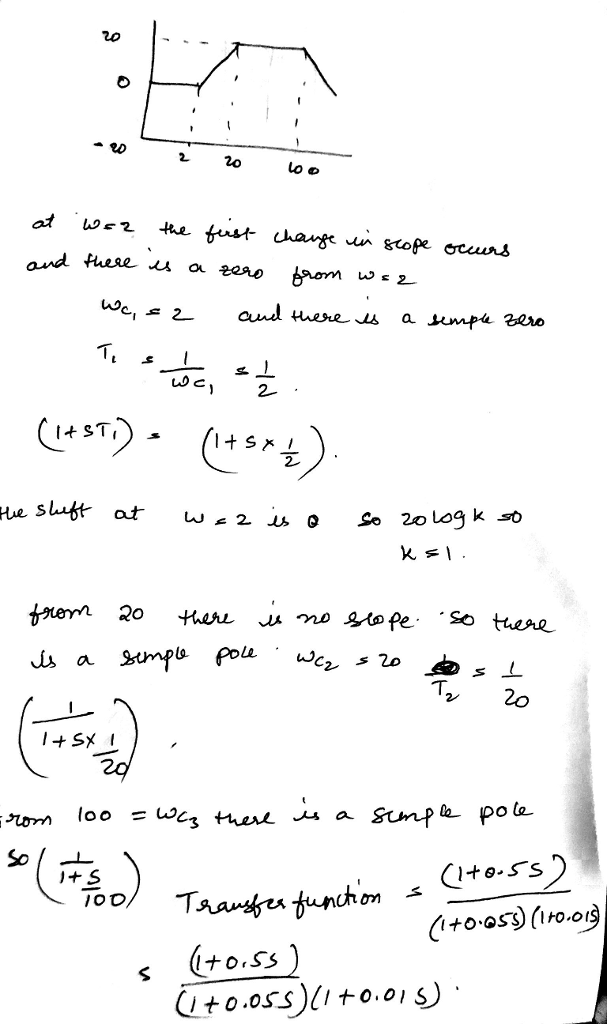#### Earn Coins

Coins can be redeemed for fabulous gifts.

Similar Homework Help Questions
• ### The Bode magnitude plot of H(o) is shown Find H(w) 0.1 10 ? (rad/s) +20 dB/decade...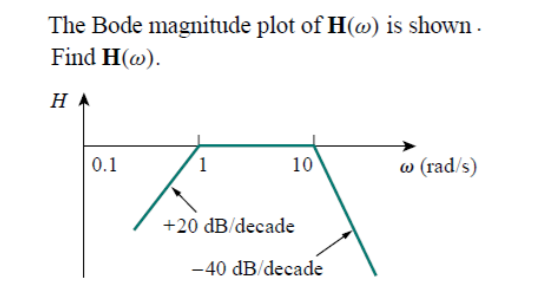The Bode magnitude plot of H(o) is shown Find H(w) 0.1 10 ? (rad/s) +20 dB/decade -40 dB/decade

• ### 12.18 Find the transfer function G(0) using the Bode magnitude plot shown below. Hw G/dB) -20...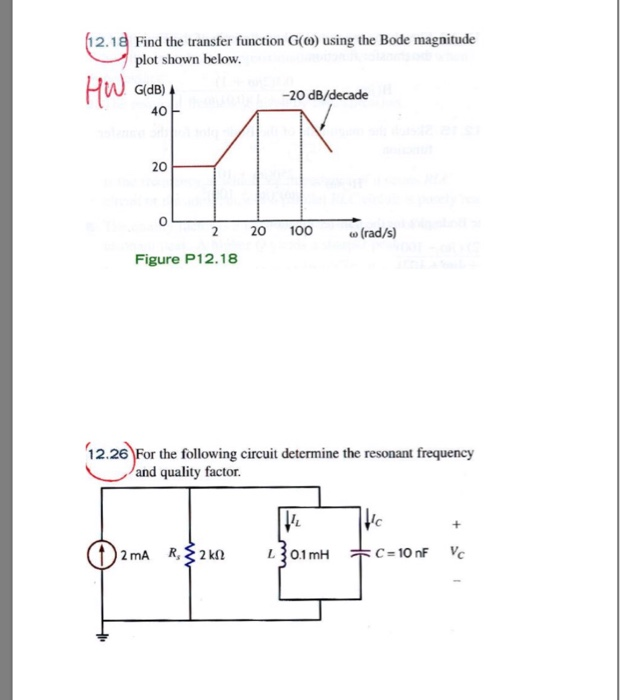12.18 Find the transfer function G(0) using the Bode magnitude plot shown below. Hw G/dB) -20 dB/decade 40 20 100 20 (rad/s) Figure P12.18 12.26 For the following circuit determine the resonant frequency and quality factor. c = 10 nF 2mA R,2k L0.1 mH c

• ### Chapter 12, Problem 12.21 (Multistep) Part 1 Correct. Sketch the magnitude characteristic of the Bode plot...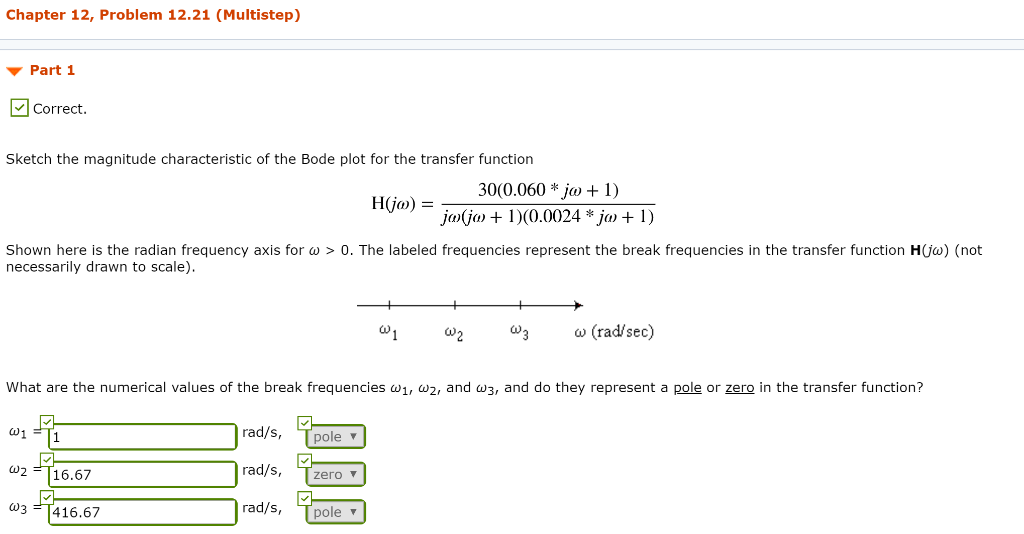Chapter 12, Problem 12.21 (Multistep) Part 1 Correct. Sketch the magnitude characteristic of the Bode plot for the transfer function 30(0.060 * jo + 1) jojo + 1)(0.0024 * ja + 1) H0) = Shown here is the radian frequency axis for w > 0. The labeled frequencies represent the break frequencies in the transfer function H(o) (not necessarily drawn to scale). -+ W + w W3 W (rad/sec) What are the numerical values of the break frequencies w1, wz,...

• ### Problem Three: Estimating a Transfer Function from a Bode Plot Based on the Bode Plot below,...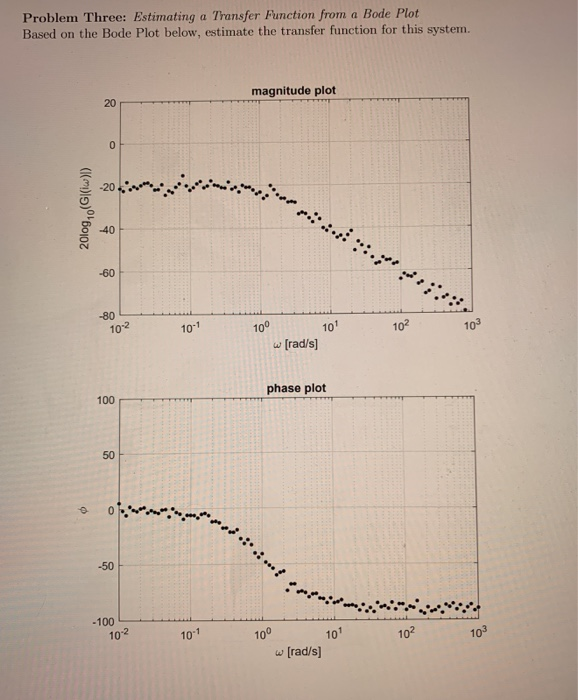Problem Three: Estimating a Transfer Function from a Bode Plot Based on the Bode Plot below, estimate the transfer function for this system. magnitude plot 20 0 2010g, ((w)) 40 -60 -80 10-2 10-1 10° 101 102 w (rad/s) phase plot 100 50 © -50 -100 102 10" 10° 102 10 101 (rad/s]

• ### A bode plot of the transfer function, GS = - 25 \$2+45+25, is shown as below....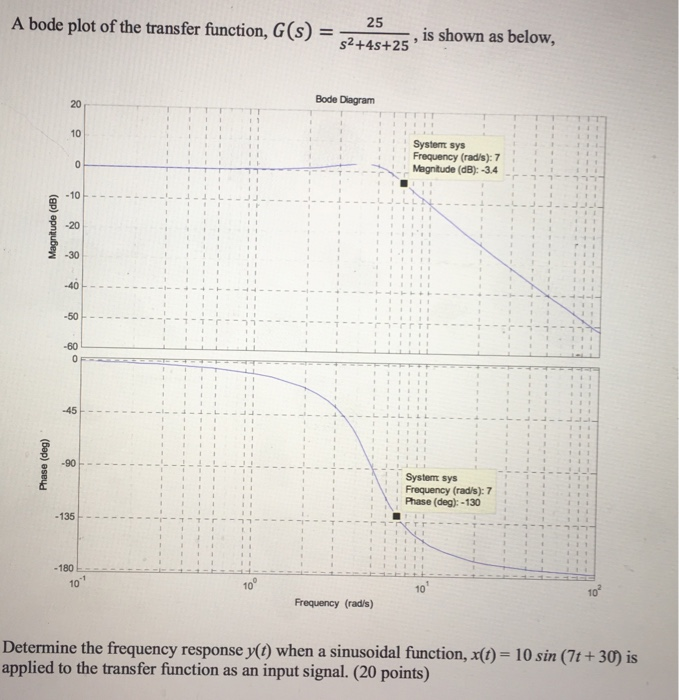A bode plot of the transfer function, GS = - 25 \$2+45+25, is shown as below. Bode Diagram System sys Frequency (rad/s): 7 Magnitude (dB): -3.4 Magnitude (dB) Phase (deg) Systemt sys Frequency (rad/s): 7 Phase (deg): - 130 - 135 - 180 10 Frequency (rad/s) Determine the frequency response y(t) when a sinusoidal function, X(t) = 10 sin (7t +30) is applied to the transfer function as an input signal. (20 points)

• ### 4. Draw the magnitude characteristic of the Bode plot of the following transfer function: 10s H...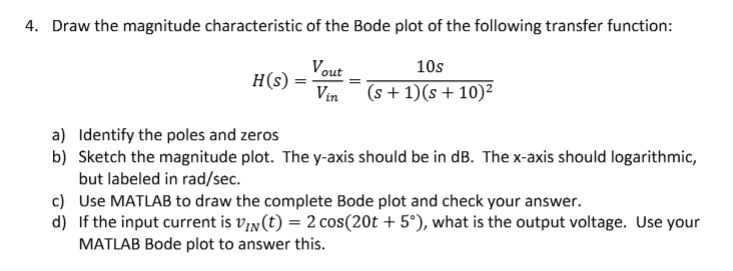4. Draw the magnitude characteristic of the Bode plot of the following transfer function: 10s H (s) =-= (s+1)(s+102 L7n Identify the poles and zeros Sketch the magnitude plot. The y-axis should be in dB. The x-axis should logarithmic, but labeled in rad/sec Use MATLAB to draw the complete Bode plot and check your answer. If the input current is un(t) = 2 cos(20t + 5°), what is the output voltage. Use your MATLAB Bode plot to answer this a)...

• ### Chapter 12, Problem 12.16 Describe the sketch of the magnitude characteristic of the Bode plot for...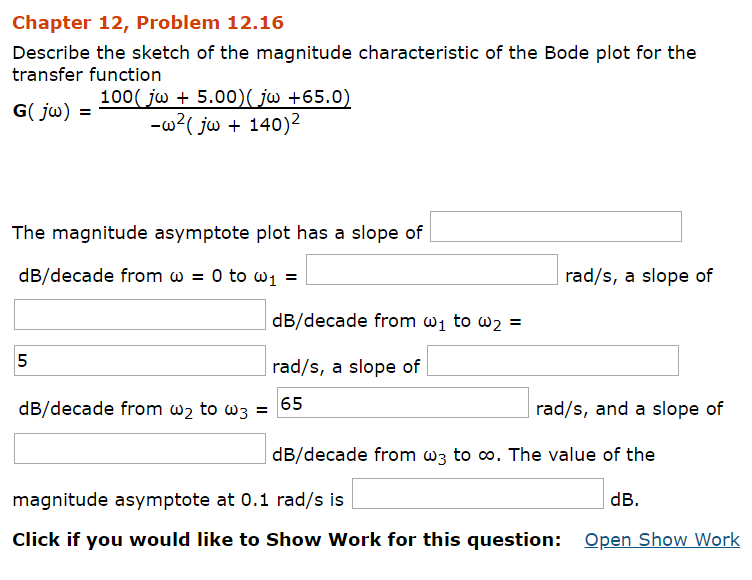Chapter 12, Problem 12.16 Describe the sketch of the magnitude characteristic of the Bode plot for the transfer function The magnitude asymptote plot has a slope of dB/decade from ω-0 to ω! rad/s, a slope of dB/decade from ω1 to ω2- 5 rad/s, a slope of dB/decade from ω2 to ω3-65 rad/s, and a slope of dB/decade from w3 to oo. The value of the magnitude asymptote at 0.1 rad/s is dB. Click if you would like to Show Work...

• ### Sketch the approximate Bode plot (magnitude and phase) of the transfer function (jw) H(W) = (w...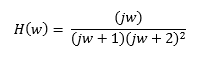Sketch the approximate Bode plot (magnitude and phase) of the transfer function (jw) H(W) = (w + 1) (w + 2)2

• ### 5. Draw the magnitude characteristic of the Bode plot of the following transfer function: H(s)10(10s +11...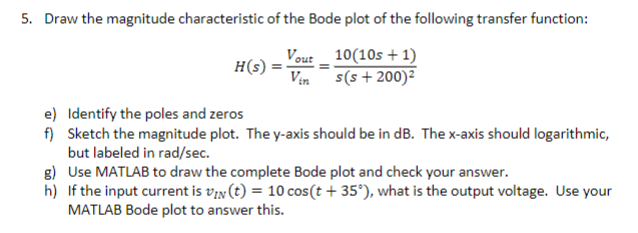5. Draw the magnitude characteristic of the Bode plot of the following transfer function: H(s)10(10s +11 (S + 200)2 Identify the poles and zeros Sketch the magnitude plot. The y-axis should be in dB. The x-axis should logarithmic, but labeled in rad/sec e) f) g) Use MATLAB to draw the complete Bode plot and check your answer. h) If the input current is VIN(t)-10 cos(t+35"),what is the output voltage. Use your MATLAB Bode plot to answer this.

• ### QUESTION #2 PLEASE 1. Derive the transfer function for the circuit shown below. Plot H(s) versus...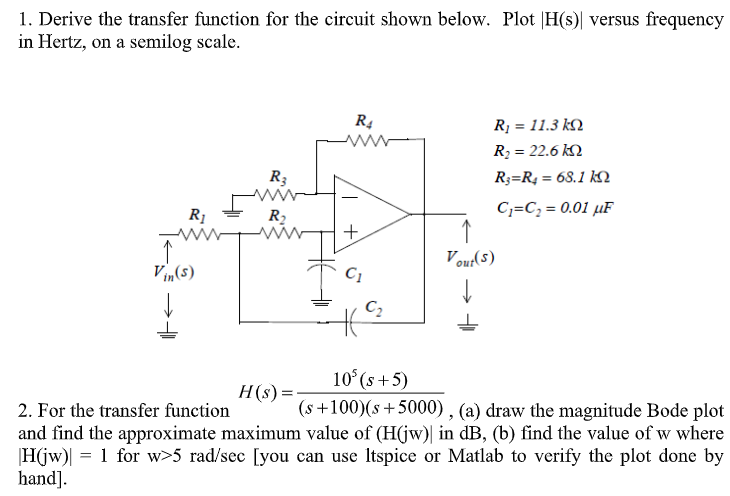QUESTION #2 PLEASE 1. Derive the transfer function for the circuit shown below. Plot H(s) versus frequency in Hertz, on a semilog scale. Ri 11.3 k Ri 22.6 k R R = 68.1 kN R3 C C 0.01 uF R2 Vout(s) Vin(s) C2 10 (s+5) H(s) = (s+100)(s5000) , (a) draw the magnitude Bode plot 2. For the transfer function and find the approximate maximum value of (H(jw) in dB, (b) find the value of w where 1 for w>5...